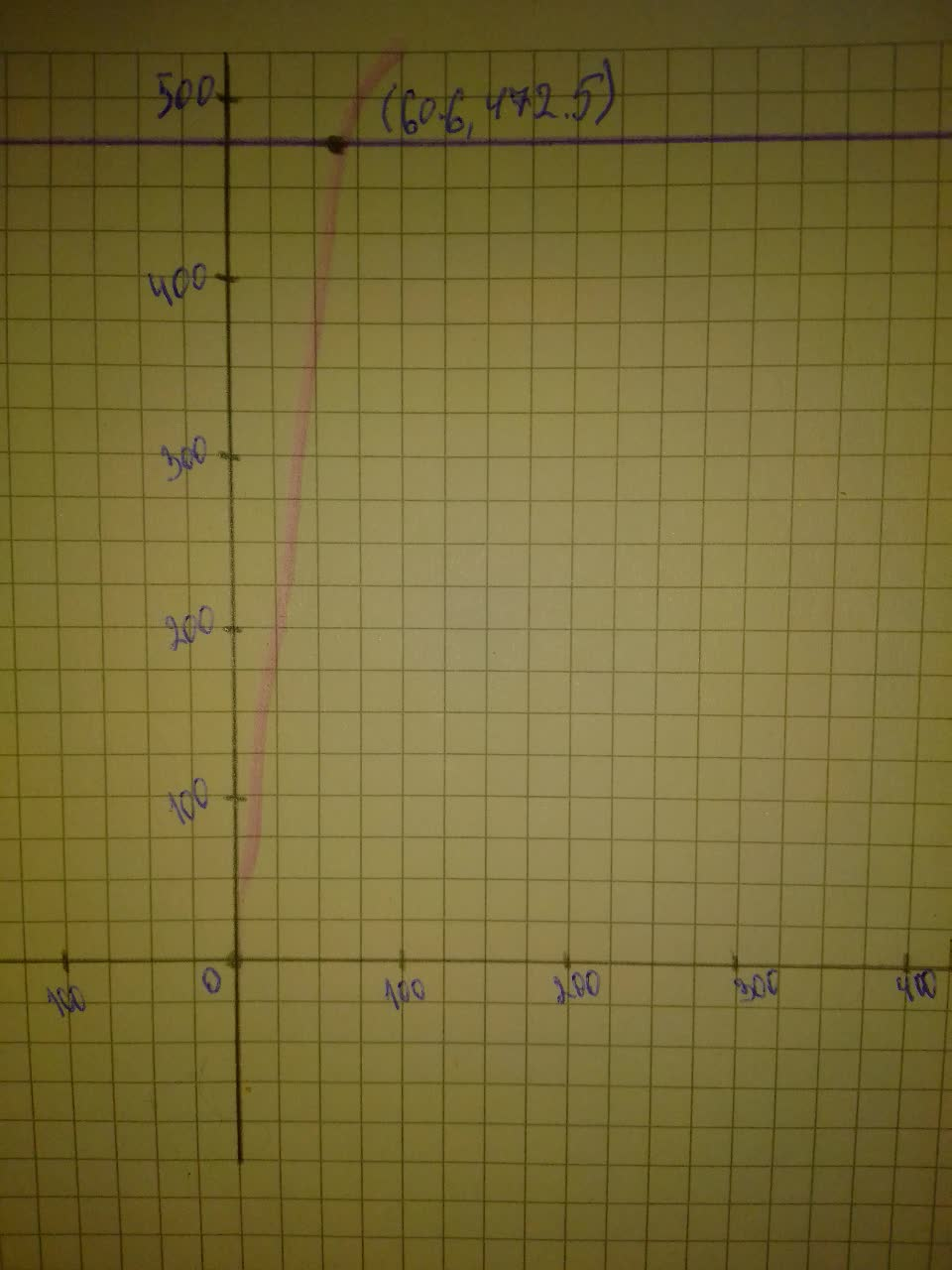# Sociologists have found that information spreads among a population at an exponential rate. Graph the function on a graphing calculator.Rui Baldwin 2021-08-03 Answered

Sociologists have found that information spreads among a population at an exponential rate. Suppose that the function $y=525\left(1-{e}^{-0.038t}\right)$ models the number of people in a town of 525 people who have heard news within t hours of its distribution. Graph the function on a graphing calculator. When will 90% of the people have heart about the grocery store opening?

You can still ask an expert for help

• Live experts 24/7
• Questions are typically answered in as fast as 30 minutes
• Personalized clear answers

Solve your problem for the price of one coffee

• Math expert for every subject
• Pay only if we can solve itliannemdh
Step 1
Determine the $90\mathrm{%}$ of the people on the town:
$=0.9×525=472.5$
then graph $y=472.5$
the x coordinate of intersection point represent the time required for the $90\mathrm{%}$ to hear the opening
conclude that $t\approx 61$ hour
Step 2